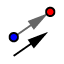# Exploring Translations in the Coordiante Plane

## DIRECTIONS:

In the applet below, 1) Construct a triangle. 2) Use the VECTOR TOOLto construct any vector whose terminal points DOES NOT lie on top of its initial point. 3) Display the label of the triangle's 3 vertices and vector you've just constructed. Use the "Name and Value" option when showing the label. 4) Use the TRANSLATE BY VECTOR TOOLto translate the triangle by the given vector.

## 5)

Suppose the coordinates of a point P = (x, y). What is the image of P under a translation by vector with components <a, b>?